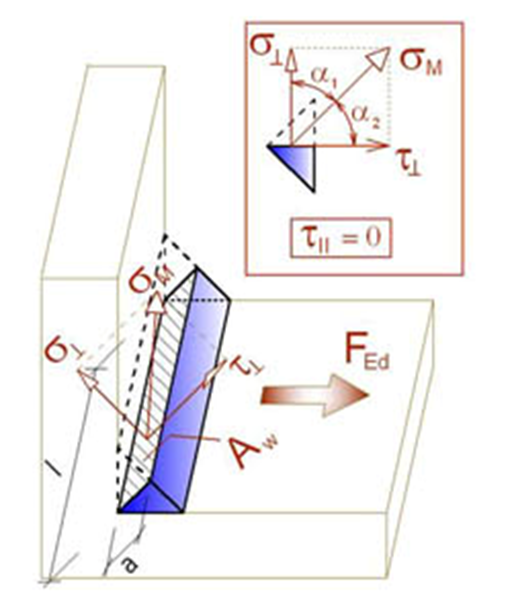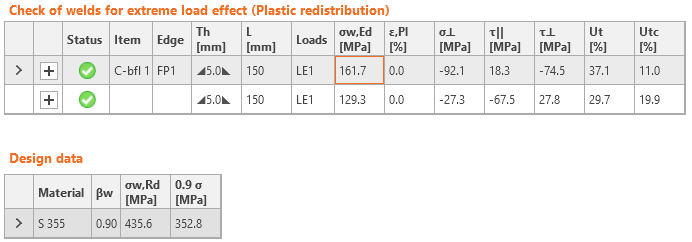# Check of welds according to Eurocode

$$Fillet welds are checked according to EN 1993-1-8. The strength of butt welds is assumed the same as the base metal and is not checked.Fillet weldsDesign resistanceThe plastic redistribution in welds is used to automatically avoid the stress singularities in weld elements, to redistribute the stress further along the weld length. The strength of the weld approximately matches the hand calculation and the stress is correctly distributed for complicated issues like welding to unstiffened flange (EN 1993-1-8 – Cl. 4.10). The stress in the throat section of a fillet weld is determined according to EN 1993-1-8 Cl. 4.5.3. Stresses are calculated from the stresses in weld element. Bending moment around the weld longitudinal axis is not taken into account.$\sigma_{w,Ed}=\sqrt{\sigma_{\perp}^2 + 3 \left ( \tau_{\perp}^2 + \tau_{\parallel}^2 \right )}$$\sigma_{w,Rd} = \frac{f_u}{\beta_w \gamma_{M2}}$Weld utilization$U_t = \min \left\{ \frac{\sigma_{{w,Ed}}}{\sigma_{w,Rd}}, \frac{\sigma_{\perp}}{0.9 f_u / {\gamma_{M2}}} \right\}$where: σw,Ed – equivalent stress in the weld σw,Rd – weld resistance βw – correlation factor (EN 1993-1-8 – Table 4.1) fu – ultimate strength, chosen as the lower of the two connected base materials or according to material chosen by user γM2 – safety factor (EN 1993-1-8 – Table 2.1; editable in Code setup) σ┴, τ┴, τ‖ – stresses in weld according to the figure below:All values required for check are printed in tables. Ut is the utilization of the most stressed element. Since plastic redistribution of stress in weld is used, it is the decisive utilization. Utc provides information about utilization along the weld length. It is the ratio of actual stress at all elements of the weld to the design resistance of the stress of the whole length of the weld.The equivalent stress in the weld diagram shows the following stress:$\sigma = \max \left \{ \frac{\sigma_{\perp}}{0.9 \beta_w}, \, \sqrt{\sigma_{\perp}^2 + 3 \tau_{\perp}^2 + 3 \tau_{\parallel}^2} \right \}$Butt weldsWelds can be specified as butt welds. Complete joint penetration is considered for butt welds, and therefore such welds are not checked.$$

## Vyzkoušejte program IDEA StatiCa

Stáhněte si zdarma zkušební verzi na 14 dní.